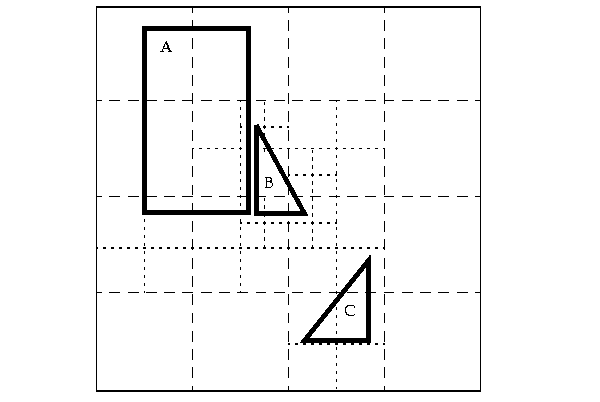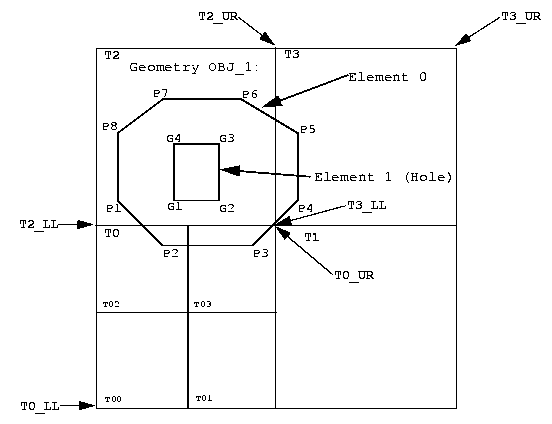# B Hybrid Indexing

Quadtree hybrid indexing uses a combination of fixed-size and variable-sized tiles for spatially indexing a layer. Variable-sized tile spatial indexing uses tiles of different sizes to approximate a geometry. For each geometry, you will have a set of fixed-size tiles that fully cover the geometry, and also a set of variable-sized tiles that fully cover the geometry.

For most applications, you should not use hybrid indexes, but should instead use quadtree fixed indexes or R-tree indexes. The rare circumstances where hybrid indexes should be considered are as follows:

• When joins are required between layers whose optimal fixed index level (SDO_LEVEL) values are significantly different (4 levels or more), it may be possible to get better performance by bringing the layer with a higher optimal SDO_LEVEL down to the lower SDO_LEVEL and adding the SDO_NUMTILES parameter to ensure adequate tiling of the layer.

The best starting value for SDO_NUMTILES in the new hybrid layer can be calculated by getting a count of the rows in the spatial index table and dividing this number by the number of rows with geometries in the layer, then rounding up. A spatial join ('QUERYTYPE=JOIN') is not a common requirement for applications, and it is comparable to a spatial cross product where each of the geometries in one layer will be compared with each of the geometries in the other layer.

• When both of the following are true for a single layer, hybrid indexing may be preferable: (1) the layer has a mixture of many geometries covering a very small area and many polygons covering a very large area; and (2) the optimal fixed tiling level for the very small geometries will result in an extremely large number of tiles to be generated for the very large geometries, causing the spatial index to grow to an unreasonable size.

If both of these conditions are true, it may be better to use the SDO_NUMTILES parameter to get coverage for the smaller geometries, while keeping the fixed tile size relatively large for the large geometries by using a smaller SDO_LEVEL value.

In Figure B-1, the variable-sized cover tiles closely approximate each geometry. This results in good selectivity. The number of variable tiles needed to cover a geometry is controlled using the SDO_NUMTILES parameter.

Figure B-1 Variable-Sized Tile Spatial IndexingDescription of the illustration sdo_hyba.gif

A variable tile is subdivided if it interacts with the geometry, and subdivision will not result in tiles that are smaller than a predetermined size. This size, or tiling resolution, is determined by a default SDO_MAXLEVEL value.

Figure B-2 illustrates how geometry OBJ_1 is approximated with hybrid indexing (SDO_LEVEL = 1 and SDO_NUMTILES = 4). These are not recommended values for SDO_LEVEL and SDO_NUMTILES; they were chosen to simplify this example. The cover tiles are stored in the SDOINDEX table as shown in Table B-1.

Figure B-2 Decomposition of the GeometryDescription of the illustration sdo_hyb2.gif

In Figure B-2, note that for simplicity the tiles have been numbered, and LL and UR indicate lower left and upper right, respectively. For example, T2_LL indicates the lower left corner of tile T2. (This designation scheme does not reflect the actual format use in Spatial.)

In Figure B-2, note which fixed-size tiles are associated with geometry OBJ_1. Only three (T0, T2, T3) of the four large tiles (T0, T1, T2, T3) generated by the tessellation actually interact with the geometry. Only those three are stored in the SDOINDEX table. In examining which variable-sized tiles are used, tile T0 shows a further tessellation to four smaller tiles, two of which (T02, T03) are used to cover a portion of the geometry. The variable-sized tiles are stored in the SDO_CODE column in the Spatial index table. The fixed-size tiles are stored in the SDO_GROUPCODE column. The spatial index structure is discussed in Section 2.5.

Table B-1 shows the tiles from Figure B-2 that are stored in the SDOINDEX table.

Table B-1 Section of the SDOINDEX Table

SDO_ROWID <RAW> SDO_CODE <RAW> SDO_MAXCODE <RAW> SDO_GROUPCODE <RAW> SDO_META <RAW>
GID_OBJ_1 T02 <binary data> T0 <binary data>
GID_OBJ_1 T03 <binary data> T0 <binary data>
GID_OBJ_1 T2 <binary data> T2 <binary data>
GID_OBJ_1 T3 <binary data> T3 <binary data>

As with the fixed-size tile model, all elements in a geometry are tessellated in one step. In a multielement geometry like OBJ_1, Element 1 (the hole shown in Figure B-2) is covered by a redundant tile (T2) from the tessellation of Element 0, but this tile is stored only once.

The SDO_TUNE package has some functions that help determine appropriate SDO_LEVEL and SDO_NUMTILES values.

## B.1 Creating a Hybrid Index

This section describes hybrid indexing, which uses both fixed-size and variable-sized tiles as a spatial indexing mechanism. For each geometry, you will have a set of fixed-size tiles that fully covers the geometry, and a set of variable-sized tiles that fully covers the geometry. The terms hybrid indexing, hybrid tiling, and hybrid tessellation are used interchangeably in this section.

To use hybrid tiling, the SDO_LEVEL and SDO_NUMTILES keywords in the PARAMETERS clause must contain valid values. Both SDO_LEVEL and SDO_NUMTILES must be greater than 1.

The SDO_NUMTILES value determines the number of variable tiles that will be used to fully cover a geometry being indexed. Typically this value is small. For points, SDO_NUMTILES is always one. For other element types, you might set SDO_NUMTILES to a value around 8. The larger the SDO_NUMTILES value, the better the tiles will approximate the geometry being covered. A larger SDO_NUMTILES value improves the selectivity of the primary filter, but it also increases the number of index entries per geometry (see Section 4.2.1.1 and Section 4.2.1.2 for a discussion of primary and secondary filters). The SDO_NUMTILES value should be larger for long, linear spatial entities, such as major highways or rivers, than for area-related spatial entities such as county or state boundaries.

The SDO_LEVEL value determines the size of the fixed tiles used to fully cover the geometry being indexed. Setting the proper SDO_LEVEL value may appear more like art than science. Performing some simple data analysis and testing puts the process back in the realm of science. One approach would be to use the SDO_TUNE.ESTIMATE_TILING_LEVEL function to determine an appropriate starting SDO_LEVEL value, and then compare the performance with slightly higher or lower values.

In Example B-1, assume that data has been loaded into a table called ROADS, and the USER_SDO_GEOM_METADATA view has an entry for ROADS.SHAPE. (Assume also that no spatial index has already been created on the ROADS.SHAPE column.) You can use the following SQL statement to create a hybrid index named ROADS_HYBRID.

Example B-1 Creating a Hybrid Index

```CREATE INDEX ROADS_HYBRID ON ROADS(SHAPE)
INDEXTYPE IS MDSYS.SPATIAL_INDEX PARAMETERS('SDO_LEVEL=6 SDO_NUMTILES=12');
```

## B.2 Tuning Considerations with Hybrid Indexes

Hybrid indexing allows indexes to be built using the tiling mechanism by specifying the SDO_LEVEL. Additionally, hybrid indexing introduces the ability to specify the minimum number of tiles to be created for each geometry during the indexing process by specifying the indexing parameter SDO_NUMTILES.

If the number of tiles created for a geometry using the SDO_LEVEL value is less than the value specified by the SDO_NUMTILES value, then the indexing process continues by creating more tiles for the geometry until the SDO_NUMTILES value has been reached. The ability to specify the minimum number of tiles for each geometry is important for a number of reasons:

• It ensures that all geometries will have at least as many index entries as the value of SDO_NUMTILES, regardless of the tiling level.

• It can reduce (as compared to fixed indexing) the space required for index data to get full indexing coverage of all geometries.

• Special performance enhancing algorithms have been coded within Spatial to make use of hybrid indexes.

If hybrid indexing is used and if the layer being indexed is point-only data, the SDO_NUMTILES value should be set to 1.